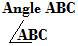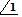## Angles

What is an angle? Or how is an angle formed?Take a look at the above figure. The figure represents an angle.

An angle is a geometrical figure formed by two non collinear rays meeting at a point.

The two rays are called the sides or arms of the angle, and the point of intersection is called vertex of the angle.

Vertex is the common end point of the two intersecting rays AB and AC.

Formation of AngleConsider the initial ray BC in the first figure above. Initial ray BC is rotated anti clockwise to reach the final position BA, as shown in the second figure.

Then an angle is said to form between the two rays BC, the initial ray and the BA, the final ray, as shown in the third figure.

An angle is a measure of rotation of the initial ray in anti clockwise direction.

Alternatively, an angle is an inclination of two different rays at a point.

Denotation of an Angle (How to name an angle)

An angle can be denoted in three different ways. They are:1. Write the symbol for angle before capital letters ABCdenotes angle formed between the two sides BA and BC with the vertex formed at B.

2. Using the angle symbolWrite the angle symbol only before the vertex ‘B’ as3. By a numeral after the angle symbolThe numeral shows the angle is formed between the two rays BC and BA.

Systems of measurement of angles:

Angles are normally measured in degrees. Degree is one of the units of measurement of angles.

If the initial ray is rotated anti clockwise a full round, then the measure of the angle is 360 degrees.

The symbol for degree is  ‘0‘.

Therefore, 360 degrees is written as 3600.

One quarter of a full revolution forms an angle whose measure is 90 degrees, written as 900. 900 is the measure of a right angle.

One half of a full revolution forms an angle whose measure is 180 degrees, written as 1800.   1800 is the measure of a straight line.

Division of Degrees into Minutes and Seconds:

One degree is equal to 60 minutes, i.e. 10 = 60′

(a single stroke at the top of a number’s right is a symbol for a minute)

and One minute equals 60 seconds, i.e. 1′ = 60′′

(two strokes at the top of a number’ right stand for seconds)

Problems

Solution:

To find the sum of the given angle measures, write them as below

60065′75"

55082′73"

Add seconds to seconds, minutes to minutes and degrees to degrees, just as we add digits of same place values of two numbers.

First add numbers in seconds as below:

75′′ + 73" = 148" = 120" + 28'" = 2′ + 28" (as 1′ = 60")

28′′ remains to be written in the seconds’ place

Carry forward 2′ to the sum of minutes’ numbers as

65′ + 82′ = 147′ + 2′

= 149′ = 120′ + 29′ = 20 + 29′ (10 = 60′)

29′ remains to be written in the minutes’ place

Carry forward  20 to the sum of degrees’ numbers as

600 + 550 = 1150 + 20 = 1170

Therefore, 60065′75" + 55082′73" = 117029′28"